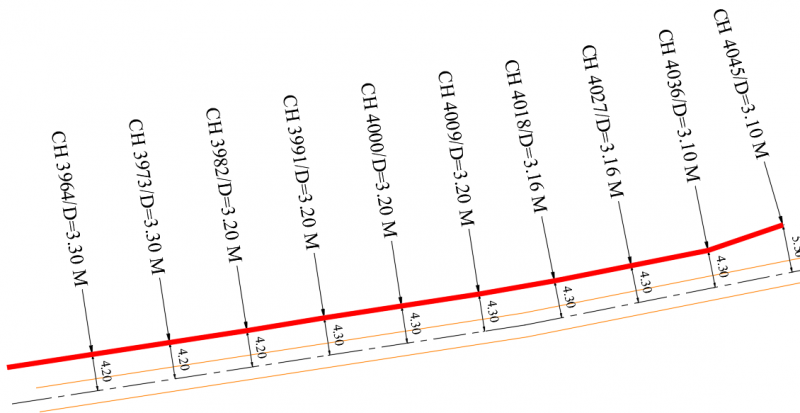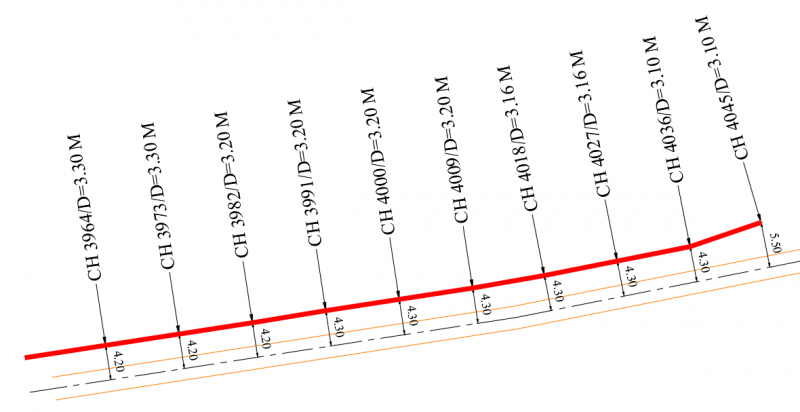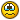# flipping multiple text at once

## Recommended PostsLooking for a lisp routine that will reverse many text mtext without moving its position, i have attached one lsp but reverse text/mtxt one by one but i want the lsp which work in bunch##### Share on other sites##### Share on other sites18 minutes ago, BIGAL said:

yes sir this the good command can u modify my lsp like this?

##### Share on other sitesPosted (edited)

Try this quicky. Mtext only, Only selects items on unlocked layers

```(defun c:rmt ( / *error* sv_lst sv_vals c_doc a_lst ss obj rot a_num i_pt)

(defun *error* ( msg )
(mapcar 'setvar sv_lst sv_vals)
(if (and c_doc (= 8 (logand 8 (getvar 'UNDOCTL)))) (vla-endundomark c_doc))
(if (not (wcmatch (strcase msg) "*BREAK*,*CANCEL*,*EXIT*")) (princ (strcat "\nOops an Error : " msg " occurred.")))
(princ)
);_end_*error*_defun

(setq sv_lst (list 'cmdecho 'osmode)
sv_vals (mapcar 'getvar sv_lst)
a_lst (list 0 9 8 7 6 5 4 3 2 1)
);end_setq

(mapcar 'setvar sv_lst '(0 0))

(if (and c_doc (= 8 (logand 8 (getvar 'UNDOCTL)))) (vla-endundomark c_doc))
(vla-startundomark c_doc)

(setq ss (ssget ":L" '((0 . "MTEXT"))))

(cond (ss
(repeat (setq cnt (sslength ss))
(setq obj (vlax-ename->vla-object (ssname ss (setq cnt (1- cnt))))
rot (vlax-get-property obj 'rotation)
a_num (vlax-get-property obj 'attachmentpoint)
i_pt (vlax-get-property obj 'insertionpoint)
)
(cond ( (< (/ pi 2.0) rot (* (/ pi 2.0) 3))
(setq a_num (nth a_num a_lst)
rot (+ pi rot)
)
(mapcar '(lambda (x y) (vlax-put-property obj x y)) (list 'rotation 'attachmentpoint 'insertionpoint) (list rot a_num i_pt))
)
);end_cond
);end_repeat
)
);end_cond

(if (and c_doc (= 8 (logand 8 (getvar 'UNDOCTL)))) (vla-endundomark c_doc))
(mapcar 'setvar sv_lst sv_vals)
(princ)
);end_defun

```

Edited by dlanorh
code correction

##### Share on other sites13 hours ago, dlanorh said:

Try this quicky. Mtext only, Only selects items on unlocked layers

Can this be made to work on DTEXT as well?

Steve

##### Share on other sitesDefine "DTEXT". This used to be what is now "TEXT" or perhaps you're refering to Dimension Text?

##### Share on other sitesPosted (edited)

Sorry. I meant single line text.

I know there are already ways to do this within Autocad, but always looking for ways to make the process a bit more efficient.

Edited by StevJ

##### Share on other sitesIn that case something similar will work on text, but not using this method as, depending on the alignment different points are used (insertion point or text alignment point. Also not every alignment has a complimentary. I'll post something in about an hour.

##### Share on other sitesThis should work for "TEXT" items. Again only on unlocked layers

```(defun rh:gbbc (obj / ll ur lst c_pt)
(if (and obj (= (type obj) 'ENAME))  (setq obj (vlax-ename->vla-object obj)))
(cond (obj
(vlax-invoke-method obj 'getboundingbox 'll 'ur)
(setq lst (mapcar 'vlax-safearray->list (list ll ur))
c_pt (mapcar '(lambda (x y) (/ (+ x y) 2.0)) (car lst) (cadr lst))
);end_setq
)
);end_cond
c_pt
);end_defun

(defun c:rt ( / *error* sv_lst sv_vals c_doc ss cnt obj rot pt)

(defun *error* ( msg )
(mapcar 'setvar sv_lst sv_vals)
(if (and c_doc (= 8 (logand 8 (getvar 'UNDOCTL)))) (vla-endundomark c_doc))
(if (not (wcmatch (strcase msg) "*BREAK*,*CANCEL*,*EXIT*")) (princ (strcat "\nOops an Error : " msg " occurred.")))
(princ)
);_end_*error*_defun

(setq sv_lst (list 'cmdecho 'osmode)
sv_vals (mapcar 'getvar sv_lst)
);end_setq

(mapcar 'setvar sv_lst '(0 0))

(if (and c_doc (= 8 (logand 8 (getvar 'UNDOCTL)))) (vla-endundomark c_doc))
(vla-startundomark c_doc)

(setq ss (ssget ":L" '((0 . "TEXT"))))

(cond (ss
(repeat (setq cnt (sslength ss))
(setq obj (vlax-ename->vla-object ent)
rot (vlax-get-property obj 'rotation)
pt (rh:gbbc obj)
);end_setq
(cond ( (< (/ pi 2.0) rot (* (/ pi 2.0) 3)) (vlax-invoke obj 'rotate pt pi)))
);end_repeat
)
);end_cond

(if (and c_doc (= 8 (logand 8 (getvar 'UNDOCTL)))) (vla-endundomark c_doc))
(mapcar 'setvar sv_lst sv_vals)
(princ)
);end_defun
```

##### Share on other sitesPosted (edited)

Unfortunately, this did not work.

I tried changing selected text to Middle Center justification with

`(command "_.justifytext" ss "" "_MC")`

just after

`(setq ss (ssget ":L" '((0 . "TEXT"))))`

so that rotation could always be  around the insertion point, which should work and would be ideal actually for single line text, but that didn't make any difference.

Steve

Edited by StevJ
spellin errers

##### Share on other sitesOn 8/10/2019 at 8:10 PM, dlanorh said:

Try this quicky. Mtext only, Only selects items on unlocked layers

```
(defun c:rmt ( / *error* sv_lst sv_vals c_doc a_lst ss obj rot a_num i_pt)

(defun *error* ( msg )
(mapcar 'setvar sv_lst sv_vals)
(if (and c_doc (= 8 (logand 8 (getvar 'UNDOCTL)))) (vla-endundomark c_doc))
(if (not (wcmatch (strcase msg) "*BREAK*,*CANCEL*,*EXIT*")) (princ (strcat "\nOops an Error : " msg " occurred.")))
(princ)
);_end_*error*_defun

(setq sv_lst (list 'cmdecho 'osmode)
sv_vals (mapcar 'getvar sv_lst)
a_lst (list 0 9 8 7 6 5 4 3 2 1)
);end_setq

(mapcar 'setvar sv_lst '(0 0))

(if (and c_doc (= 8 (logand 8 (getvar 'UNDOCTL)))) (vla-endundomark c_doc))
(vla-startundomark c_doc)

(setq ss (ssget ":L" '((0 . "MTEXT"))))

(cond (ss
(repeat (setq cnt (sslength ss))
(setq obj (vlax-ename->vla-object (ssname ss (setq cnt (1- cnt))))
rot (vlax-get-property obj 'rotation)
a_num (vlax-get-property obj 'attachmentpoint)
i_pt (vlax-get-property obj 'insertionpoint)
)
(cond ( (< (/ pi 2.0) rot (* (/ pi 2.0) 3))
(setq a_num (nth a_num a_lst)
rot (+ pi rot)
)
(mapcar '(lambda (x y) (vlax-put-property obj x y)) (list 'rotation 'attachmentpoint 'insertionpoint) (list rot a_num i_pt))
)
);end_cond
);end_repeat
)
);end_cond

(if (and c_doc (= 8 (logand 8 (getvar 'UNDOCTL)))) (vla-endundomark c_doc))
(mapcar 'setvar sv_lst sv_vals)
(princ)
);end_defun

```

not working....sir

##### Share on other sitesPosted (edited)

not working....sir

It worked when I tested it on the drawing  you attached. Any error messages?

Edited by dlanorh

##### Share on other sitesPosted (edited)
2 hours ago, StevJ said:

Unfortunately, this did not work.

I tried changing selected text to Middle Center justification with

```
(command "_.justifytext" ss "" "_MC")```

just after

```
(setq ss (ssget ":L" '((0 . "TEXT"))))```

so that rotation could always be  around the insertion point, which should work and would be ideal actually for single line text, but that didn't make any difference.

Steve

Just noticed an incorrect line. I seemed to have edited something across all documents in the editor, as opposed to the one I was working on.Try this

```(defun rh:gbbc (obj / ll ur lst c_pt)
(if (and obj (= (type obj) 'ENAME))  (setq obj (vlax-ename->vla-object obj)))
(cond (obj
(vlax-invoke-method obj 'getboundingbox 'll 'ur)
(setq lst (mapcar 'vlax-safearray->list (list ll ur))
c_pt (mapcar '(lambda (x y) (/ (+ x y) 2.0)) (car lst) (cadr lst))
);end_setq
)
);end_cond
c_pt
);end_defun

(defun c:rt ( / *error* sv_lst sv_vals c_doc ss cnt obj rot pt)

(defun *error* ( msg )
(mapcar 'setvar sv_lst sv_vals)
(if (and c_doc (= 8 (logand 8 (getvar 'UNDOCTL)))) (vla-endundomark c_doc))
(if (not (wcmatch (strcase msg) "*BREAK*,*CANCEL*,*EXIT*")) (princ (strcat "\nOops an Error : " msg " occurred.")))
(princ)
);_end_*error*_defun

(setq sv_lst (list 'cmdecho 'osmode)
sv_vals (mapcar 'getvar sv_lst)
);end_setq

(mapcar 'setvar sv_lst '(0 0))

(if (and c_doc (= 8 (logand 8 (getvar 'UNDOCTL)))) (vla-endundomark c_doc))
(vla-startundomark c_doc)

(setq ss (ssget ":L" '((0 . "TEXT"))))

(cond (ss
(repeat (setq cnt (sslength ss))
(setq obj (vlax-ename->vla-object (ssname ss (setq cnt (1- cnt))))
rot (vlax-get-property obj 'rotation)
pt (rh:gbbc obj)
);end_setq
(cond ( (< (/ pi 2.0) rot (* (/ pi 2.0) 3)) (vlax-invoke obj 'rotate pt pi)))
);end_repeat
)
);end_cond

(if (and c_doc (= 8 (logand 8 (getvar 'UNDOCTL)))) (vla-endundomark c_doc))
(mapcar 'setvar sv_lst sv_vals)
(princ)
);end_defun```

You don't need to justify the text, the rh:gbbc sub finds the centre of the bounding box and uses that.

Edited by dlanorh

##### Share on other sitesPosted (edited)
7 hours ago, dlanorh said:

Just noticed an incorrect line. I seemed to have edited something across all documents in the editor, as opposed to the one I was working on.Try this

```
(defun rh:gbbc (obj / ll ur lst c_pt)
(if (and obj (= (type obj) 'ENAME))  (setq obj (vlax-ename->vla-object obj)))
(cond (obj
(vlax-invoke-method obj 'getboundingbox 'll 'ur)
(setq lst (mapcar 'vlax-safearray->list (list ll ur))
c_pt (mapcar '(lambda (x y) (/ (+ x y) 2.0)) (car lst) (cadr lst))
);end_setq
)
);end_cond
c_pt
);end_defun

(defun c:rt ( / *error* sv_lst sv_vals c_doc ss cnt obj rot pt)

(defun *error* ( msg )
(mapcar 'setvar sv_lst sv_vals)
(if (and c_doc (= 8 (logand 8 (getvar 'UNDOCTL)))) (vla-endundomark c_doc))
(if (not (wcmatch (strcase msg) "*BREAK*,*CANCEL*,*EXIT*")) (princ (strcat "\nOops an Error : " msg " occurred.")))
(princ)
);_end_*error*_defun

(setq sv_lst (list 'cmdecho 'osmode)
sv_vals (mapcar 'getvar sv_lst)
);end_setq

(mapcar 'setvar sv_lst '(0 0))

(if (and c_doc (= 8 (logand 8 (getvar 'UNDOCTL)))) (vla-endundomark c_doc))
(vla-startundomark c_doc)

(setq ss (ssget ":L" '((0 . "TEXT"))))

(cond (ss
(repeat (setq cnt (sslength ss))
(setq obj (vlax-ename->vla-object (ssname ss (setq cnt (1- cnt))))
rot (vlax-get-property obj 'rotation)
pt (rh:gbbc obj)
);end_setq
(cond ( (< (/ pi 2.0) rot (* (/ pi 2.0) 3)) (vlax-invoke obj 'rotate pt pi)))
);end_repeat
)
);end_cond

(if (and c_doc (= 8 (logand 8 (getvar 'UNDOCTL)))) (vla-endundomark c_doc))
(mapcar 'setvar sv_lst sv_vals)
(princ)
);end_defun```

You don't need to justify the text, the rh:gbbc sub finds the centre of the bounding box and uses that.

sir command????? i was wrote rt but also asking for select object

##### Share on other sitessir command????? i was wrote rt but also asking for select object

This is a different version to work with "TEXT",  Not Leader "MTEXT" as in your attached drawing, and it was posted to @StevJ in response to a request from him.

I have rechecked the lisp I attached in my post to you, and it still works with the drawing you posted. It is a stand alone lisp for Leader MText, as altering your lisp to work with a selection sets (multiple objects) would entail rewriting most of a large multi-purpose lisp file. The lisp will flip any "unreadable" leader MText (i.e. text with a rotation angle >90 <270) through 180 to make it readable. The command for the lisp I attached to you is "rmt"

##### Share on other sites19 hours ago, dlanorh said:

Just noticed an incorrect line. I seemed to have edited something across all documents in the editor, as opposed to the one I was working on.Try this

Unfortunately it still will not work.

I tried to understand the program and maybe try to figure out why, but I have difficulties with the vla- and vlax- parts.

If I could find a way to easily select lines of TEXT and convert them to separate and individual MTEXT (Express Tools is not my friend for this), your RMT program works well and would do the job handily, but I haven't found a way to do that either (yet). Still looking, though.

Steve

##### Share on other sitesnot working....sir

thank you very much sir.....

##### Share on other sitesPosted (edited)
On 8/10/2019 at 8:10 PM, dlanorh said:

Try this quicky. Mtext only, Only selects items on unlocked layers

```
(defun c:rmt ( / *error* sv_lst sv_vals c_doc a_lst ss obj rot a_num i_pt)

(defun *error* ( msg )
(mapcar 'setvar sv_lst sv_vals)
(if (and c_doc (= 8 (logand 8 (getvar 'UNDOCTL)))) (vla-endundomark c_doc))
(if (not (wcmatch (strcase msg) "*BREAK*,*CANCEL*,*EXIT*")) (princ (strcat "\nOops an Error : " msg " occurred.")))
(princ)
);_end_*error*_defun

(setq sv_lst (list 'cmdecho 'osmode)
sv_vals (mapcar 'getvar sv_lst)
a_lst (list 0 9 8 7 6 5 4 3 2 1)
);end_setq

(mapcar 'setvar sv_lst '(0 0))

(if (and c_doc (= 8 (logand 8 (getvar 'UNDOCTL)))) (vla-endundomark c_doc))
(vla-startundomark c_doc)

(setq ss (ssget ":L" '((0 . "MTEXT"))))

(cond (ss
(repeat (setq cnt (sslength ss))
(setq obj (vlax-ename->vla-object (ssname ss (setq cnt (1- cnt))))
rot (vlax-get-property obj 'rotation)
a_num (vlax-get-property obj 'attachmentpoint)
i_pt (vlax-get-property obj 'insertionpoint)
)
(cond ( (< (/ pi 2.0) rot (* (/ pi 2.0) 3))
(setq a_num (nth a_num a_lst)
rot (+ pi rot)
)
(mapcar '(lambda (x y) (vlax-put-property obj x y)) (list 'rotation 'attachmentpoint 'insertionpoint) (list rot a_num i_pt))
)
);end_cond
);end_repeat
)
);end_cond

(if (and c_doc (= 8 (logand 8 (getvar 'UNDOCTL)))) (vla-endundomark c_doc))
(mapcar 'setvar sv_lst sv_vals)
(princ)
);end_defun

```

one problem found lsp was somewhere working and somewhere not.

##### Share on other sitesone problem found lsp was somewhere working and somewhere not. and not working on dtext

##### Share on other sites8 hours ago, StevJ said:

Unfortunately it still will not work.

I tried to understand the program and maybe try to figure out why, but I have difficulties with the vla- and vlax- parts.

If I could find a way to easily select lines of TEXT and convert them to separate and individual MTEXT (Express Tools is not my friend for this), your RMT program works well and would do the job handily, but I haven't found a way to do that either (yet). Still looking, though.

Steve

Post an example drawing (2012). This is probably something simple I'm overlooking, as it works on my system.

Attached is a documented version if it helps, and my test drawing (2012). I have updated the lisp by inserting a debug "alert" for null selection set (nothing selected) as well as a prompt.

When prompted for the text entities select everything by crossing, and only three items should be highlighted, everything else should be ignored.

## Join the conversation

You can post now and register later. If you have an account, sign in now to post with your account.
Note: Your post will require moderator approval before it will be visible.×   Pasted as rich text.   Paste as plain text instead

Only 75 emoji are allowed.